# Grids and grid properties#

This section offers an overview of the different methods and functions available for the Petrel Grid object subcategories.

## Grids#

Check out the API documentation to view a detailed description of all the functions and methods available for the Grid objects

Let’s look at an example on how to retrieve all the grids from our Petrel project by using the .grids property. This property returns all the grids from the project in a dictionary where the keys represent the path of the grid within the Petrel input tree and the value represents the name of the grid:

:

from cegalprizm.pythontool import PetrelConnection
petrel = PetrelConnection()

all_grids=petrel.grids
print(all_grids)

{'Models/Gullfaks2004/Gullfaks (Make Horizon)': Grid(petrel_name="Gullfaks (Make Horizon)"), 'Models/Final model/Training': Grid(petrel_name="Training"), 'Models/Gullfaks2004/Gullfaks Final (DC)': Grid(petrel_name="Gullfaks Final (DC)")}


Using a for loop, we can iterate through the dictionary and print out all the grid names:

:

for grid in petrel.grids:
print(grid.petrel_name)

Gullfaks (Make Horizon)
Gullfaks Final (DC)
Training


Let’s select a specific grid (Gullfaks Final (DC)). Specific grids can be accessed by using the unique id or by using the path. Petrel allows equal object names, so the path is not guaranteed to be unique. In cases of equal paths, use the unique id instead of the path to look up:

:

a_grids_id_and_path = list(all_grids.keys())
grid_path = a_grids_id_and_path
grid = petrel.grids[grid_path]
print(grid.petrel_name)

Gullfaks Final (DC)

[ ]:



:

for el in grid.segments:
print (el)

Segment(petrel_name="Segment 1")
Segment(petrel_name="Segment 2")
Segment(petrel_name="Segment 3")
Segment(petrel_name="Segment 4")
Segment(petrel_name="Segment 5")
Segment(petrel_name="Segment 6")
Segment(petrel_name="Segment 7")
Segment(petrel_name="Segment 8")
Segment(petrel_name="Segment 9")
Segment(petrel_name="Segment 10")
Segment(petrel_name="Segment 11")
Segment(petrel_name="Segment 12")
Segment(petrel_name="Segment 13")
Segment(petrel_name="Segment 14")
Segment(petrel_name="Segment 15")
Segment(petrel_name="Segment 16")
Segment(petrel_name="Segment 17")
Segment(petrel_name="Segment 18")
Segment(petrel_name="Segment 19")
Segment(petrel_name="Segment 20")


We can obtain the size of the grid using the .extent property, which returns the number of cells in the i, j and k directions:

:

extent = grid.extent
print(extent)

Extent(i=85, j=88, k=88)


The .coords_extent returns the extent of the grid in world-coordinates (Xmin, Xmax, Ymin, Ymax, Zmin, Zmax):

:

coord_extent = grid.coords_extent
print(f'x: {coord_extent.x_axis}')
print(f'y: {coord_extent.y_axis}')
print(f'z: {coord_extent.z_axis}')

x: AxisExtent(min=450658.280000, max=459028.480000)
y: AxisExtent(min=6780231.600000, max=6790163.160000)
z: AxisExtent(min=-2366.750000, max=-1751.230000)


Using a for loop and the .properties property we can iterate through the available properties within the selected grid and print them out: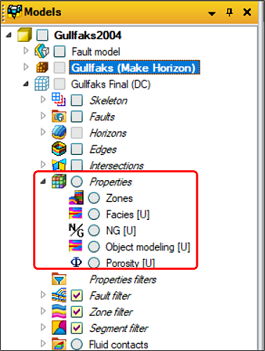:

for prop in grid.properties:
print(prop)

GridProperty(petrel_name="NG")
GridProperty(petrel_name="Porosity")
GridDiscreteProperty(petrel_name="Zones")
GridDiscreteProperty(petrel_name="Facies")
GridDiscreteProperty(petrel_name="Object modeling")


Using the .indices() and .position() functions we can obtain the centre of a cell with its coresponding indices. First, we assign the indices of a cell to the cell_indices variable. For the indices parameters, we are using the i,j,k extent of the cell and then use floor division to get i,j,k of the centre of the cell. Once we have the i,j,k of the centre, we can then use the .position() function to get the XYZ position of the cell centre based on the provided i,j,k parameters:

:

from cegalprizm.pythontool.primitives import Indices
from cegalprizm.pythontool.primitives import Point

cell_indices = Indices(i = extent.i//2, j = extent.j//2, k = extent.k//2)
cell_center_position = grid.position(cell_indices.i, cell_indices.j, cell_indices.k)
print(f'{cell_indices}: {cell_center_position}')

Indices(i=42, j=44, k=44): Point(x=454926.72, y=6784597.32, z=-2034.18)


Alternatively, we can obtain the indices of a cell at a specified XYZ position:

:

pos = cell_center_position
cell_indices_at_pos = grid.indices(pos.x, pos.y, pos.z)
print(f'{pos}: {cell_indices_at_pos}')

Point(x=454926.72, y=6784597.32, z=-2034.18): Indices(i=42, j=44, k=44)


To get the vertices position of cell at a specified ijk position, we can use the .vertices() function. Use the vertices_unchecked() function if you do not wish to spend time checking if the cell is defined. In that case, the method will return a list of undefined points.

:

vertices_positions = grid.vertices(cell_indices.i, cell_indices.j, cell_indices.k)
print(f'{cell_indices}: {vertices_positions} ')

Indices(i=42, j=44, k=44): [Point(x=454898.0417201763, y=6784539.240852976, z=-2045.9384765625), Point(x=454893.4293825538, y=6784646.287928432, z=-2042.516357421875), Point(x=454964.516370921, y=6784655.51934186, z=-2025.9385986328125), Point(x=454958.8456553315, y=6784548.233269478, z=-2026.3314208984375), Point(x=454893.67790898, y=6784539.2028891565, z=-2043.9384765625), Point(x=454889.500361688, y=6784646.247008894, z=-2040.516357421875), Point(x=454960.70478615444, y=6784655.546750721, z=-2023.9385986328125), Point(x=454955.02119874046, y=6784548.276951542, z=-2024.3314208984375)]


With the release of Python Tool Pro version 2.2, it is now possible to retrieve zone and segment information of a 3D grid model.

You can access the segments in your 3D grid using the .segments property which will return an iterable collection of the segments for the grid:

:

grid.segments

:

Segments(grid="Grid(petrel_name="Gullfaks Final (DC)")")


Using a for loop we can iterate through all the segments or alternatively we can generate a list of them using the .list() function:

:

for sg in grid.segments:
print(sg)

segments_list = list(grid.segments)
print(segments_list)

Segment(petrel_name="Segment 1")
Segment(petrel_name="Segment 2")
Segment(petrel_name="Segment 3")
Segment(petrel_name="Segment 4")
Segment(petrel_name="Segment 5")
Segment(petrel_name="Segment 6")
Segment(petrel_name="Segment 7")
Segment(petrel_name="Segment 8")
Segment(petrel_name="Segment 9")
Segment(petrel_name="Segment 10")
Segment(petrel_name="Segment 11")
Segment(petrel_name="Segment 12")
Segment(petrel_name="Segment 13")
Segment(petrel_name="Segment 14")
Segment(petrel_name="Segment 15")
Segment(petrel_name="Segment 16")
Segment(petrel_name="Segment 17")
Segment(petrel_name="Segment 18")
Segment(petrel_name="Segment 19")
Segment(petrel_name="Segment 20")
[Segment(petrel_name="Segment 1"), Segment(petrel_name="Segment 2"), Segment(petrel_name="Segment 3"), Segment(petrel_name="Segment 4"), Segment(petrel_name="Segment 5"), Segment(petrel_name="Segment 6"), Segment(petrel_name="Segment 7"), Segment(petrel_name="Segment 8"), Segment(petrel_name="Segment 9"), Segment(petrel_name="Segment 10"), Segment(petrel_name="Segment 11"), Segment(petrel_name="Segment 12"), Segment(petrel_name="Segment 13"), Segment(petrel_name="Segment 14"), Segment(petrel_name="Segment 15"), Segment(petrel_name="Segment 16"), Segment(petrel_name="Segment 17"), Segment(petrel_name="Segment 18"), Segment(petrel_name="Segment 19"), Segment(petrel_name="Segment 20")]


We can assign a segment to a variable using list slicing:

:

seg1=segments_list
seg1

:

Segment(petrel_name="Segment 1")


The .cells property will return the indices of the cells belonging to this segment. In the example bellow we are only returning the first 10 values ([0:10]):

:

seg1.cells[0:10]

:

[Indices(i=5, j=47, k=None),
Indices(i=5, j=46, k=None),
Indices(i=5, j=45, k=None),
Indices(i=5, j=44, k=None),
Indices(i=6, j=48, k=None),
Indices(i=6, j=47, k=None),
Indices(i=6, j=46, k=None),
Indices(i=6, j=45, k=None),
Indices(i=6, j=44, k=None),
Indices(i=6, j=43, k=None)]


The .is_cell_inside() function checks if a cell is inside a segment at a particular i and j position. It returns True if a cell exists and false if not :

:

seg1.is_cell_inside(seg1.cells)

:

True


To retrieve the statistics of a particular segment you can use the .retrieve_stats() function:

:

seg1.retrieve_stats()

:

{'Number of cells': '641',
'Z Max': '-1882.63',
'X Delta': '3673.54999999999',
'Z Delta': '544.8',
'Long Delta': '0.0696037777777776',
'Z Min': '-2427.43',
'Lat Delta': '0.0454362500000016',
'Long Min': '2.08947588888889',
'Y Max': '6788807.26',
'X Max': '454780.81',
'Lat Min': '61.1840995555556',
'Lat Max': '61.2295358055556',
'X Min': '451107.26',
'Segment area': '5.32036E+6 m2',
'Y Min': '6783794.61',
'Long Max': '2.15907966666667',
'Y Delta': '5012.64999999944'}


The .grid property retunrns the grid associated with the particular segment:

:

seg1.grid

:

Grid(petrel_name="Gullfaks Final (DC)")


You can access the zones in your 3D grid using the .zones property which will return an iterable collection of the zones for the grid:

:

grid.zones

:

Zones(grid="Grid(petrel_name="Gullfaks Final (DC)")")


Using a for loop we can iterate through all the zones or alternatively we can generate a list of them using the .list() function:

:

for zn in grid.zones:
print(zn)

zones_list = list(grid.zones)
print(zones_list)

Zone(petrel_name="Zone 1 Sh")
Zone(petrel_name="Tarbert")
Zone(petrel_name="Ness")
[Zone(petrel_name="Zone 1 Sh"), Zone(petrel_name="Tarbert"), Zone(petrel_name="Ness")]


We can assign a segment to a variable using list slicing:

:

Ness=zones_list
Ness

:

Zone(petrel_name="Ness")


The .base_k property will return the bottom geological horizon index in the 3D grid:

:

Ness.base_k

:

87


The .top_k property will return the top geological horizon index in the 3D grid:

:

Ness.top_k

:

48


To retrieve the statistics of a particular zone you can use the .retrieve_stats() function:

:

Ness.retrieve_stats()

:

{'Number of geological layers covered': '40',
'Y Min': '6780231.6',
'Nodes (nI x nJ)': '86 x 89',
'Cells (nI x nJ)': '85 x 88',
'Y Delta': '9917.8200000003',
'Total number of 3D cells (Simbox)': '299200',
'Lat Max': '61.2420517777778',
'X Min': '450941.36',
'Long Max': '2.23883397222222',
'Z Min': '0',
'Z Max': '189.51',
'': '',
'X Max': '459028.48',
'Z Delta': '189.51',
'Y Max': '6790149.42',
'Total number of 2D cells': '7480',
'Average Zinc (along pillar)': '4.40745578',
'Bottom geological horizon index in 3D grid': '89',
'Lat Delta': '0.0899549999999962',
'Total number of 2D nodes': '7654',
'Top geological horizon index in 3D grid': '49',
'Lat Min': '61.1520967777778',
'X Delta': '8087.12',
'Long Min': '2.08603736111111',
'Covers geological layers': '49 - 88',
'Long Delta': '0.152796611111111'}


The .zones property will retrun an iterable collection of all the sub-zones associated to the selected zone: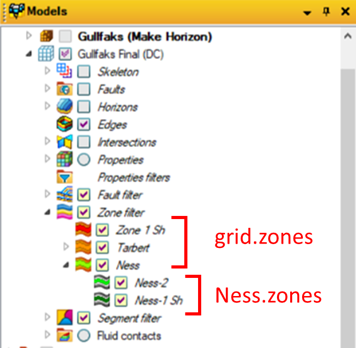:

Ness.zones

:

Zones(zone="Zone(petrel_name="Ness")")


We can assign the sub-zone to a variable using list slicing:

:

Ness2=zone1.zones
Ness2

:

Zone(petrel_name="Ness-2")


As we seen above, the IJ pairs of segments and the top and base k of zones can be accessed and retrun. They can olso be matched to the IJK values of a (grid property) chunk dataframe thus allowing users to retrieve, update or create new values for specific zones and/or segments.

## Grid properties#

Check out the API documentation to view a detailed description of all the functions and methods available for the Grid properties:

To retrieve all the grid properties from our Petrel project we can use the .grid_properties property. This property returns all the properties from all the grids within the project in a dictionary where the keys represent the path of the grid properties within the Petrel input tree and the value represents the name of the property:

:

all_grids_prop=petrel.grid_properties
print(all_grids_prop)

{'Models/Gullfaks2004/Gullfaks Final (DC)/Properties/NG': GridProperty(petrel_name="NG"), 'Models/Final model/Training/Properties/Conditioned porosity': GridProperty(petrel_name="Conditioned porosity"), 'Models/Final model/Training/Properties/Porosity': GridProperty(petrel_name="Porosity"), 'Models/Gullfaks2004/Gullfaks Final (DC)/Properties/Porosity': GridProperty(petrel_name="Porosity")}


Using a for loop, we can iterate through the dictionary and print out all the grid properties names. For accessing discrete properties, we have to use the .discrete_grid_properties property. Furthermore, we can also return the grid name for every property using the .grid property:

:

for grid_prop in petrel.grid_properties:
print('Property name:' , grid_prop.petrel_name, "  |  Grid name:", grid_prop.grid.petrel_name)
for disc_grid_prop in petrel.discrete_grid_properties:
print('Discrete property name:', disc_grid_prop.petrel_name, "  |  Grid name:",disc_grid_prop.grid.petrel_name )

Property name: NG   |  Grid name: Gullfaks Final (DC)
Property name: Conditioned porosity   |  Grid name: Training
Property name: Porosity   |  Grid name: Training
Property name: Porosity   |  Grid name: Gullfaks Final (DC)
Discrete property name: Facies   |  Grid name: Training
Discrete property name: Object modeling   |  Grid name: Gullfaks Final (DC)
Discrete property name: Layers   |  Grid name: Training
Discrete property name: Object modeling   |  Grid name: Training
Discrete property name: Zones   |  Grid name: Gullfaks Final (DC)
Discrete property name: Facies   |  Grid name: Gullfaks Final (DC)


Let’s select the Porosity and Facies properties from the Gullfaks Final (DC) grid. First, we save all the paths of the continuous properties to a list, then we can slice through the list and assign the selected value to the Porosity variable.

To get the Facies property we have to repeat the same process by first defining the dictionary that contains the paths and values for all the discrete properties, and then by selecting the property using list slicing on the list of paths:

:

grid_prop_paths = list(all_grids_prop.keys())
Porosity_prop_path = grid_prop_paths
Porosity = petrel.grid_properties[Porosity_prop_path]

all_discrete_grid_prop= petrel.discrete_grid_properties
discrete_grid_prop_path=list(all_discrete_grid_prop.keys())
Facies_prop_path= discrete_grid_prop_path
Facies = petrel.discrete_grid_properties[Facies_prop_path]

print(Porosity.petrel_name)
print(Facies.petrel_name)

Porosity
Facies


To get the cell indices of the values which have been upscaled, we can use the .upscaled_cells property. In the following example, we are using a counter and a for loop to return just the first ten values (as there are hundreds of cells):

:

c=0
for el in Porosity.upscaled_cells:
if c<10:
print (el)
c+=1

Indices(i=57, j=77, k=0)
Indices(i=58, j=77, k=0)
Indices(i=7, j=74, k=0)
Indices(i=8, j=74, k=0)
Indices(i=27, j=61, k=0)
Indices(i=28, j=61, k=0)
Indices(i=72, j=46, k=0)
Indices(i=73, j=46, k=0)
Indices(i=14, j=44, k=0)
Indices(i=61, j=37, k=0)


The .template property returns the Petrel template for the object as a string. If no template is available, it will return an empty string. In Petrel, for the grid property example, the template can be found by accessing the setting of a property. Similarly, using the .unit_symbol property, we can access the unit for any object associated with a certain template. In Petrel, you can access this data by propping up the global settings for the selected property template.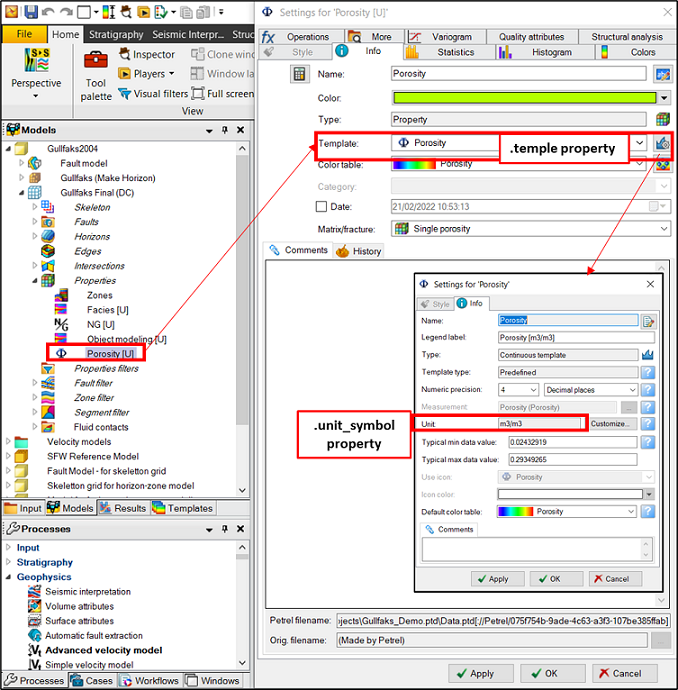:

Porosity.template

:

'Porosity'

:

Porosity.unit_symbol

:

'm3/m3'


The .discrete_codes property returns a dictionary of discrete codes as keys and the associated facies as values. Changes to this dictionary will not be persisted or affect any Petrel objects: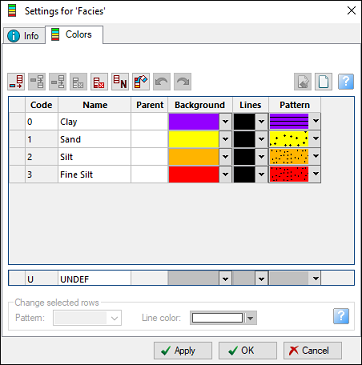:

Facies.discrete_codes

:

{0: 'Blocky', 1: 'Coarsening upwards', 2: 'Fining upwards'}


Using the .layers() function, we can set all the property values to a specific value across a range of k-slices. In the following example, we replace all the Porosity values with the value of 0.9 for k-slices between k=10 and k=19. Before iterating through each k-slice in the for loop, we set the readonly value of the Porosity property to False, so we can modify the values. If we don’t specify the range, the for loop will replace all of the values. The results are written to Petrel in real time: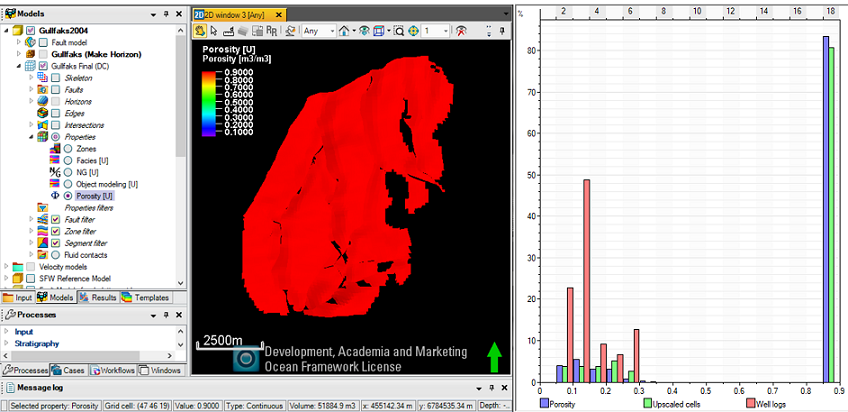:

Porosity.readonly=False
for layer in Porosity.layers(range(10,20)):
layer.set(0.9)


Similarly, we can set specific values across a range of i and j slices using the .columns() function: